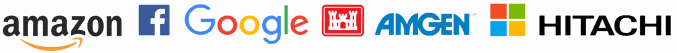# Applied linear algebra

## Linear algebra is everywhere

Nearly everything interesting depends on more than one input, and the simplest way several inputs can relate to each other is linearly. So linear algebra is a part of understanding most interesting systems.

## Even where it’s not obvious

Problems that aren’t stated in terms of linear algebra still have linear algebra in the background. As we’ve written about elsewhere, everything boils down to linear algebra.

It may seem questionable to say that linear algebra is at the heart of applied math because it’s linear. What about nonlinear applications, such as nonlinear PDEs? Nonlinear differential equations lead to nonlinear algebraic equations when discretized. But these nonlinear systems are solved via iterations of linear systems, so we’re back to linear algebra.## Matrices are big

Linear systems tend to be very large in application. If a system depends on N inputs, you can expect matrices of size at least N × N to show up. But if these inputs are continuous, each input can turn into a large number of discrete points. For example, suppose you want to know what’s happening in a cubic meter of space. If you want to track things at a resolution of one centimeter then you have not just 3 inputs but 1003 = 1,000,000 inputs. Discretizing a differential equation over this cube creates a system of a million equations in a million unknowns.

## Matrices are sparse

Linear systems also tend to be sparse, described by a matrix with mostly zero entries. In the example above, a solution every point in the cube depends on every other point in the cube, but not directly. The solution at each point directly depends only on that point’s neighbors. Simply having a large number of zeros in a matrix doesn’t necessarily help, but often there’s a pattern to the zeros that can be exploited. In this example, the geometric structure of the cube creates to an algebraic structure in the corresponding matrices.

As another example, consider Google search results. The rank of each page depends on the number and rank of pages linking to it, so conceptually Google is manipulating a matrix with a row and column for every page they track. The vast majority of this matrix is zeros since no page links to more than a few of the 4.5 billion pages out there.

## Numerical linear algebra algorithms

Linear algebra at scale is not just a larger version of what you learn in high school. Because matrices are so large and so sparse, even storing and accessing big sparse matrices requires some cleverness.

Since linear algebra is at the heart of so many problems, linear solvers are often in the inner loop of a larger application, and so efficiency is critical. Numerical linear algebra algorithms have been improving steadily for decades. For very large problems, algorithms have done more than Moore’s law to reduce computation costs.

Of course you need accuracy as well as efficiency; it doesn’t matter how quickly you can compute a wrong answer. Two ways of computing the same thing that are algebraically equivalent in theory may not be equivalent at all when carried out by a real computer. One may be very accurate and the other useless. A great deal of scholarship and practical experience has gone into understanding linear algebra algorithms deeply, not just how they behave in theory but also how they behave in actual computer hardware.

Linear algebra software packages like LAPACK are very mature. Countless experts have devoted their careers to refining these implementations. Most people have no business trying to improve on such software. For most of us, the challenge is to formulate our applications well and to choose the best algorithms, not to re-implement fundamental methods.

## Need help?

If you need some help with an application of linear algebra to your business, please let us know.

Trusted consultants to some of the world’s leading companies